Search Adaface test library by skills or roles
⌘ K

# Adaface Sample Pseudo Code Questions

Here are some sample Pseudo Code questions from our premium questions library (10273 non-googleable questions).

### Skills

#### Accounting

🧐 Question

Medium

Loop Large Value
Solve
```                                    Consider the following pseudo code.
```
```                                    What does foo() return for very large value of B (Choose closest option)
```

Options:

• A^B
• e^A
• ln(1+A)
• A^A

Medium

Loops Output Estimation
Time Complexity
Solve
```                                    What does sample(number) return in following pseudo code? (Select the closest approximation)
```

Options:

• number^2
• number*log(number)
• number*(log(number))^2
• number*(log(log(number)))
• number^3

Easy

Pseudo code output
Arrays
Solve
```                                    Consider the following pseudo code:
```
```                                    Which of the following statements are true about function goo()

I) If b is not in A, goo will run into an infinite loop
II) if b is not in A, goo will return -1
III) goo() will always return maximum element in A
IV) goo() is similar to a binary search
```

Options:

• A^B
• e^A
• ln(1+A)
• A^A
🧐 Question🔧 Skill

Medium

Loop Large Value
2 mins
Pseudo Code
Solve

Medium

Loops Output Estimation
Time Complexity
2 mins
Pseudo Code
Solve

Easy

Pseudo code output
Arrays
2 mins
Pseudo Code
Solve
🧐 Question🔧 Skill💪 Difficulty⌛ Time
Loop Large Value
Pseudo Code
Medium2 minsSolve
Loops Output Estimation
Time Complexity
Pseudo Code
Medium2 minsSolve
Pseudo code output
Arrays
Pseudo Code
Easy2 minsSolve

## Adaface questions are trusted by enterprises globally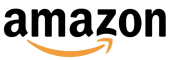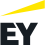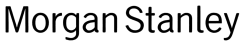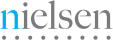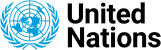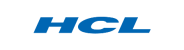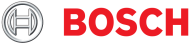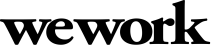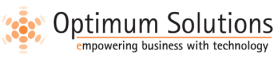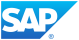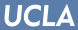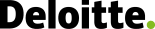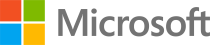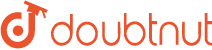We evaluated several of their competitors and found Adaface to be the most compelling. Great default library of questions that are designed to test for fit rather than memorization of algorithms.

Swayam Narain, CTO, AffableJoin 1200+ companies in 75+ countries.
Try the most candidate friendly skills assessment tool today.